# Algorithms used for Microspectroscopy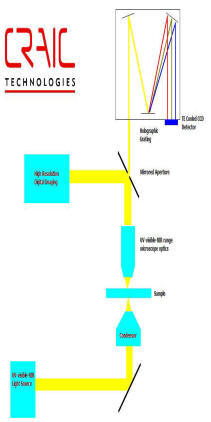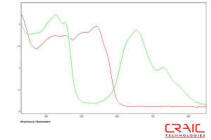Transmittance
Transmittance calculates a transmission spectrum from a sample and reference single-beam spectra.

From Two Single-Beam Spectra a transmission spectrum using the following formula: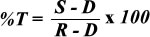where R is the single beam spectrum of the reference material, S is the single beam spectrum of the sample and D is the dark counts of the system.

From Absorbance Data a transmission spectrum can be generated using the following formula: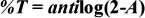where A is the absorbance value. This is a calculation from data that has already been collected.

Absorbance
Absorbance calculates an absorption spectrum from a sample and reference single-beam spectra or from a transmission spectrum.

From Two Single-Beam Spectra an absorption spectrum can be generated using the following formula:where R is the single beam spectrum of the reference material, S is the single beam spectrum of the sample and D is the dark counts of the system.

From Transmission Data an absorption spectrum can be generated using the following formula:where %T is the percent transmittance value. This is a calculation from data that has already been collected.

Reflectance
Reflectance calculates a reflectance spectrum from a sample and reference single-beam spectra or from an log 1/R spectrum.

From Two Single-Beam Spectra a reflectance spectrum can be generated using the following formula: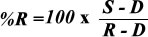where R is the single beam spectrum of the reference material, S is the single beam spectrum of the sample and D is the dark counts of the system.